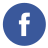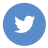### How can I check if a string is a valid number in javascript?

javascript

You can check if a string is a valid number in JavaScript by using the isNaN() function or the Number() function.

The isNaN() function returns true if the argument is not a number, and false otherwise. It can be used like this:

``````let myString = "123";
if (isNaN(myString)) {
console.log("Not a number");
} else {
console.log("Is a number");
}``````

Alternatively, you can use the Number() function to attempt to convert the string to a number. If the string is not a valid number, Number() will return NaN. You can use this fact to check if a string is a valid number like this:

``````let myString = "123";
if (isNaN(Number(myString))) {
console.log("Not a number");
} else {
console.log("Is a number");
}``````

Note that the Number() function will also convert strings that represent valid numbers (e.g. “123”) to their numeric values (e.g. 123), so be careful when using this approach.